# C/C++ program to make a simple calculator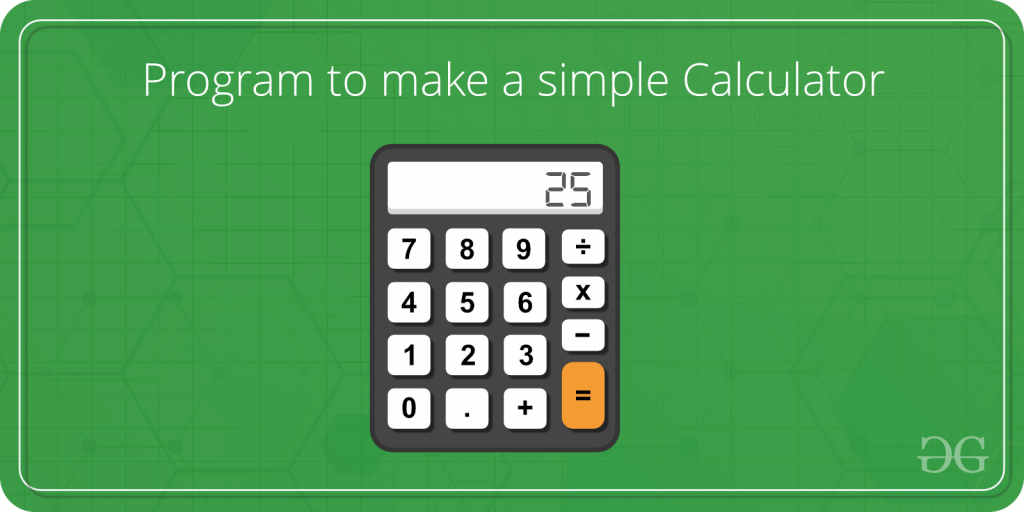Calculators are widely used device nowadays. It makes calculations easier and faster. Calculators are used to everyone in daily life. A simple calculator can be made using a C++ program which is able to add, subtract, multiply and divide, two operands entered by the user. The switch and break statement is used to create a calculator.

Program:

 `// C++ program to create calculator using ` `// switch statement ` `#include ` `using` `namespace` `std; ` ` `  `// Main program ` `main() ` `{ ` `    ``char` `op; ` `    ``float` `num1, num2; ` ` `  `    ``// It allows user to enter operator i.e. +, -, *, / ` `    ``cin >> op; ` ` `  `    ``// It allow user to enter the operands ` `    ``cin >> num1 >> num2; ` ` `  `    ``// Switch statement begins ` `    ``switch` `(op) { ` `         `  `        ``// If user enter +  ` `        ``case` `'+'``: ` `            ``cout << num1 + num2; ` `            ``break``; ` `         `  `        ``// If user enter -  ` `        ``case` `'-'``: ` `            ``cout << num1 - num2; ` `            ``break``; ` `         `  `        ``// If user enter * ` `        ``case` `'*'``: ` `            ``cout << num1 * num2; ` `            ``break``; ` `         `  `        ``// If user enter / ` `        ``case` `'/'``: ` `            ``cout << num1 / num2; ` `            ``break``; ` `         `  `        ``// If the operator is other than +, -, * or /,  ` `        ``// error message will display ` `        ``default``: ` `            ``cout << ``"Error! operator is not correct"``; ` `            ``break``; ` `    ``} ``// switch statement ends ` ` `  `    ``return` `0;  ` `} `

Output:

• Click on the run ide button, another tab will be open with web address
“https://ide.geeksforgeeks.org/index.php”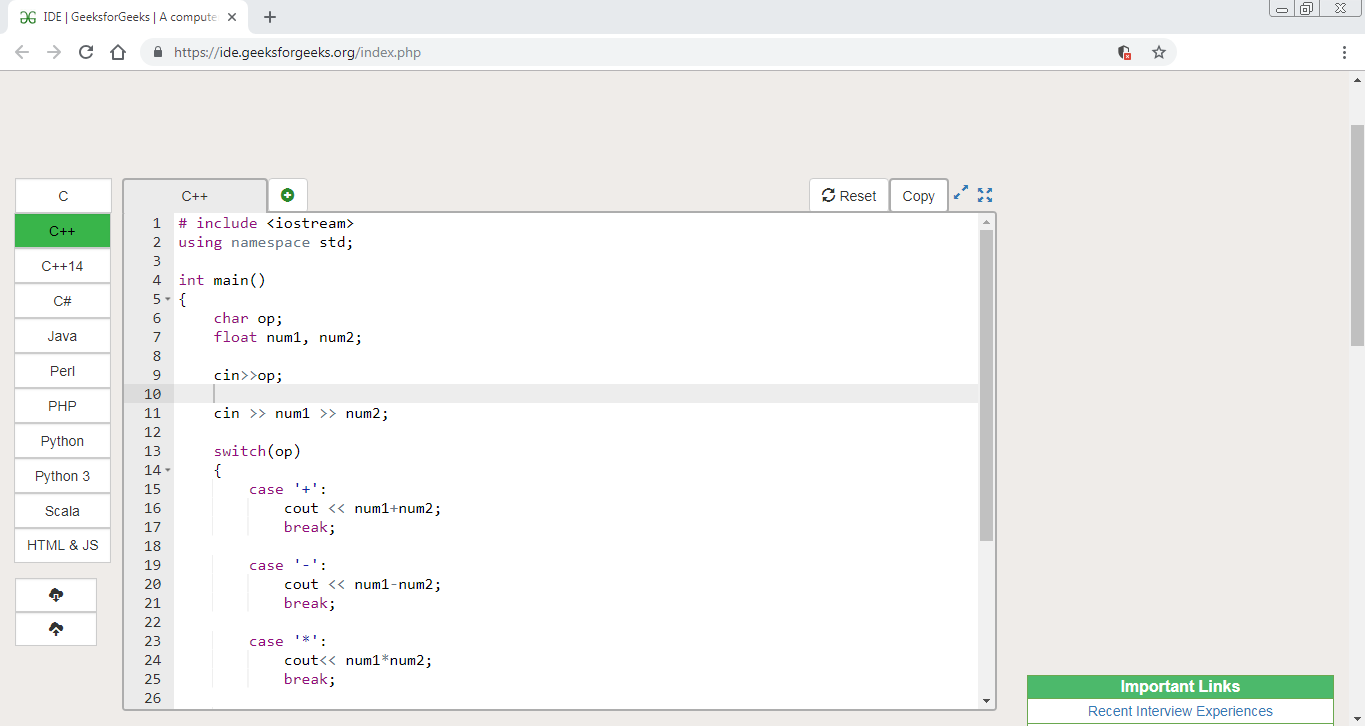• Put the input in the input box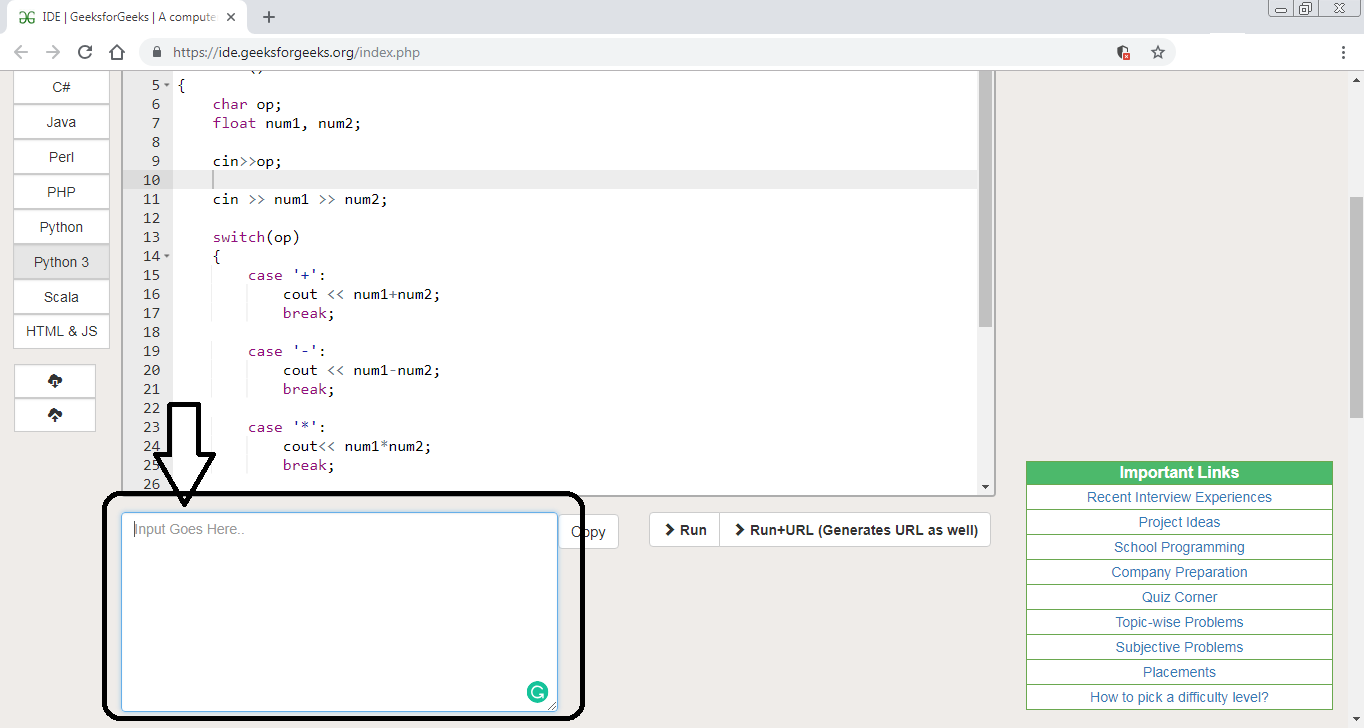• Enter the arithmetic operator (i.e.: either +, -, * or /) then, enter two operands on which need to perform the calculation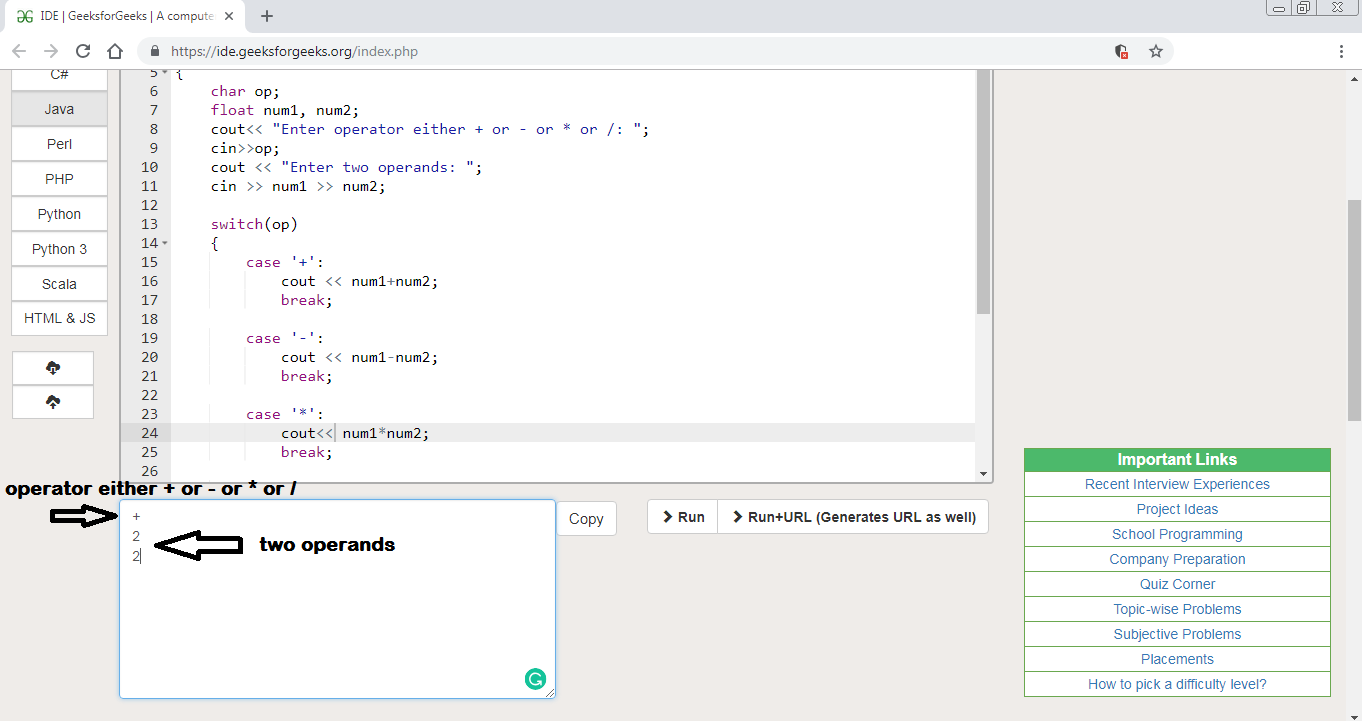• Click on the run button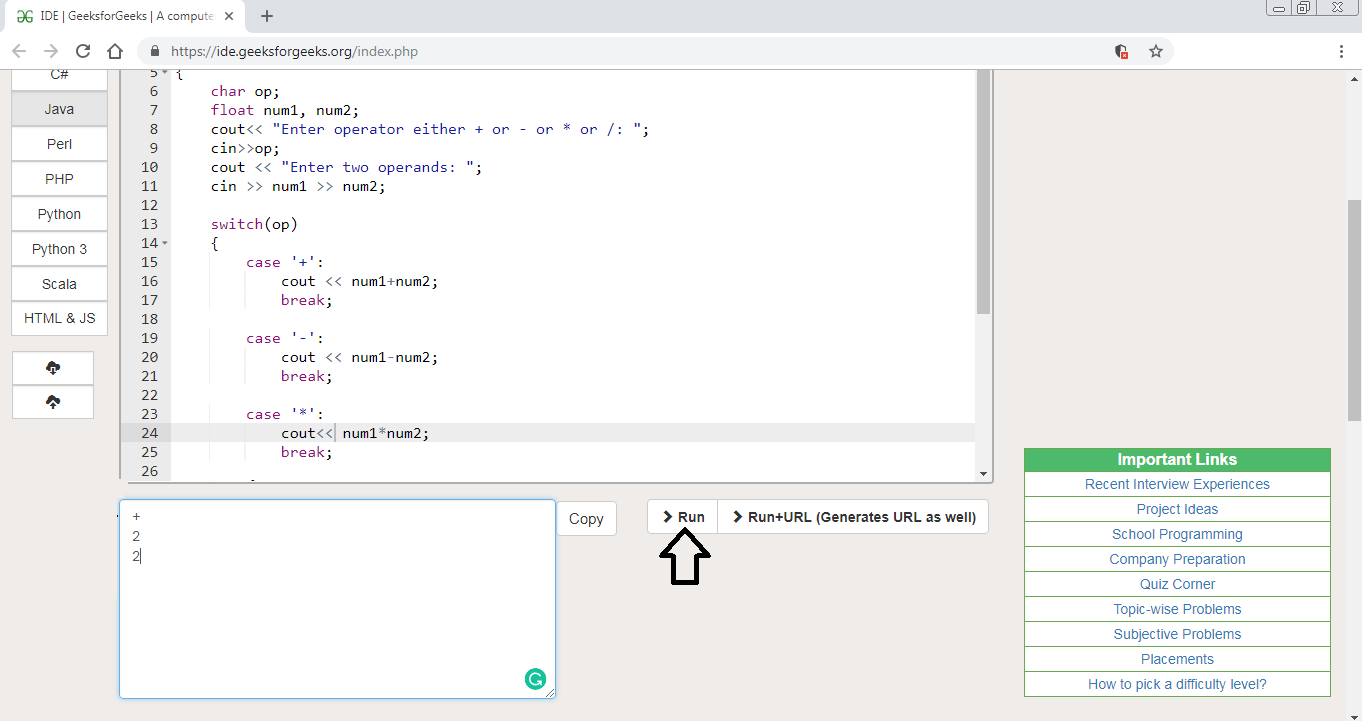• Then output box will appear with output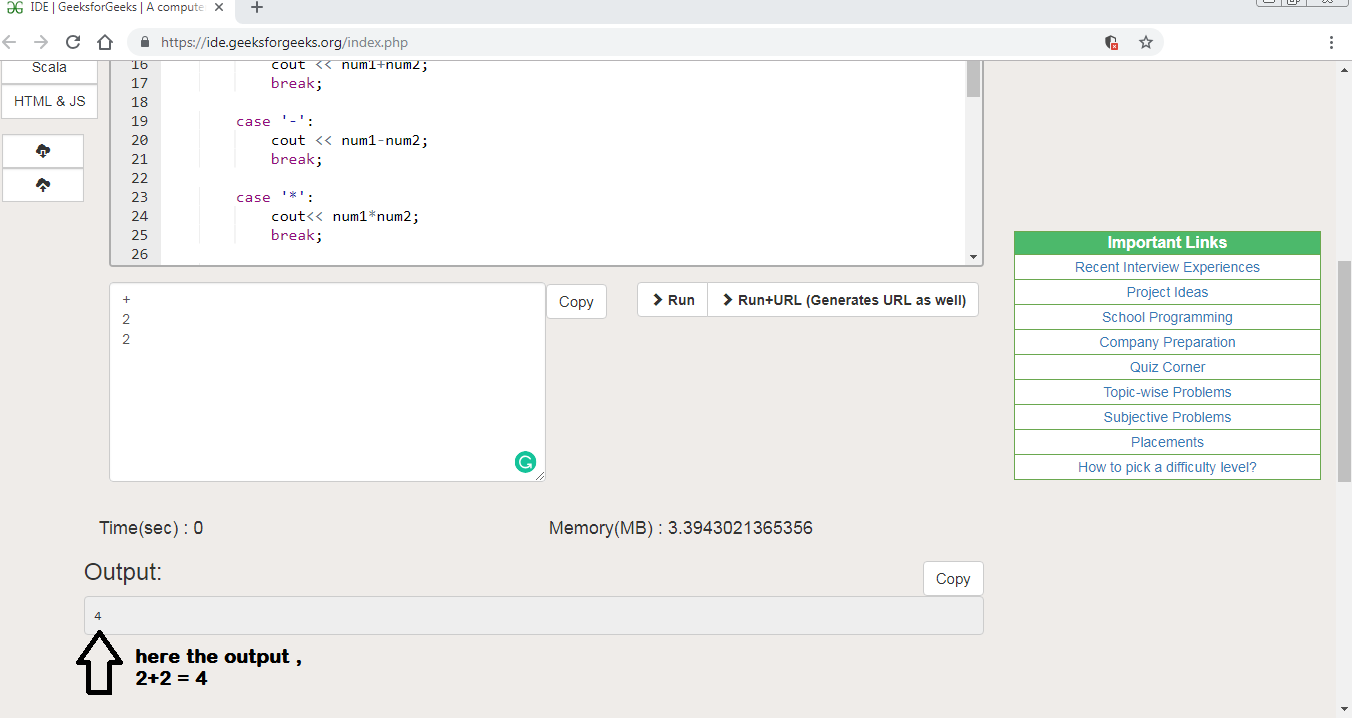My Personal Notes arrow_drop_upCheck out this Author's contributed articles.

If you like GeeksforGeeks and would like to contribute, you can also write an article using contribute.geeksforgeeks.org or mail your article to contribute@geeksforgeeks.org. See your article appearing on the GeeksforGeeks main page and help other Geeks.

Please Improve this article if you find anything incorrect by clicking on the "Improve Article" button below.